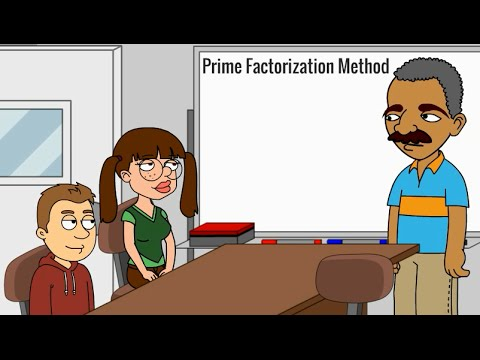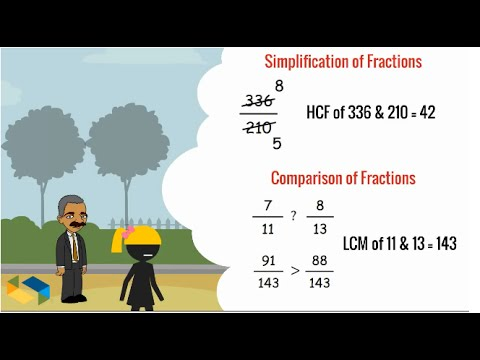## Factorisation, HCF and LCM

Subject: Compulsory Maths

#### Overview

HCF is the highest number that is the greatest thing for simplifying fractions.The smallest positive number that the multiple of two or more numbers is LCM.

#### Highest Common Factor(HCF)

HCF is the highest number that is the greatest thing for simplifying fractions. For example, the factors common to 12 and 3 are 2,3.

$\therefore$ HCF of 12 and 8 is 3.

1. HCF of monomial expressions
We can find the HCF of the given monomial expressions just by taking the common variable with the least power. The HCF of the numerical coefficient is obtained as like in the case of arithmetic. For example,
Find the HCF of x4y2 and x2y4
1st expression = x4y2
2nd expression = x2y4
$\therefore$ HCF = x2y2
2. HCF of polynomial Expression
We can find the HCF of polynomials by factorizing them. For example,
Find HCF of ax - bx and a2 - b2
1st expression = ax - bx= x(a-b)
2nd expression = a2 - b2 = (a-b)(a+b)
$\therefore$ = (a-b)

Lowest Common Multiple (LCM)

The smallest positive number that the multiple of two or more numbers is LCM. For example, the LCM of a2 and a3 is a3.

1. Lowest common factor of monomial expressions
We can find the LCM of the given monomial expressions just by taking the common variable with the highest power. For examples,
1sr expression = ax2
2nd expression = a2x2
$\therefore$ a2x2
2. LCM of polynomial expressions
To find LCM of polynomial expressions . We should factorize them. Then the product of a common factors and remaining factors is the LCM of the given expressions. For examples,
Find the L.C.M of ax2 + ax and a2x2 + a2x
1st expression = ax2 + ax = ax(x+1)
2nd expression = a2x2 + a2x = a2x(x+1)
$\therefore$ LCM = a2x(x+1)
##### Things to remember
• We can find the HCF of the given monomial expressions just by taking the common variable with the least power.
• We can find the HCF of polynomials by factorizing them.
• We can find the LCM of the given monomial expressions just by taking the common variable with the highest power.
• To find LCM of polynomial expressions . We should factorize them. Then the product of a common factors and remaining factors is the LCM of the given expressions.
• It includes every relationship which established among the people.
• There can be more than one community in a society. Community smaller than society.
• It is a network of social relationships which cannot see or touched.
• common interests and common objectives are not necessary for society.
##### Videos for Factorisation, HCF and LCM##### HCF LCM by Prime Factorization Method, Learn basics and key##### LCM, HCF - Learn basics, fundamentals with real life practical

Solution:

1st expression = x4y2

2nd expression = x2y4

$\therefore$ HCF = x2y2

Solution:

1st expression = 4ab2

= 2×2ab2

2nd expression = 6a2b3

= 2×3a2b3

$\therefore$ HCF = 2ab2

Solution:

1st expression = ax - bx = x(a-b)

2nd expression = a2 -b2 = (a+b)(a-b)

$\therefore$ HCF = (a-b)

Solution:

1st expression = x2 + xy + zx + yz = x(x+y) + z(x+y) = (x+y) (x+z)

2nd expression = x2 - y2 = (x+y) (x-y)

$\therefore$ HCF = (x+y)

Solution:

1st expression = ax2

2nd expression = a2x2

$\therefore$ LCM = a2x2

Solution:

1st expression = 4x3y3

= 2×2x3y3

2nd expression = 6xy

= 2×3xy

$\therefore$ LCM = 2×2×3 x3y3

= 12x3y3

Solution:

1st expression = ax2 + ax = ax(x+1)

2nd expression = a2x2 + a2x = a2x(x+1)

$\therefore$ LCM = a2x(x+1)